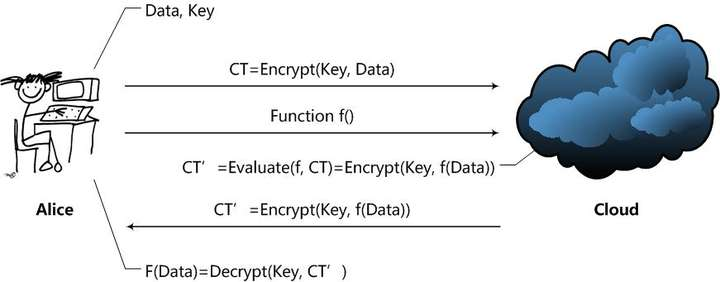# 同态加密算法

## 二、分类

• 加法同态：$f(A)+f(B)=f(A+B)$ $\Rightarrow$ 支持加减法运算
• 乘法同态：$f(A)×f(B)=f(A×B)$ $\Rightarrow$ 支持乘除法运算
• 全同态加密：同时满足加法同态和乘法同态。$\Rightarrow$ 支持加减乘除、多项式求值、指数、对数、三角函数。

• RSA 算法 —— 乘法同态
• Paillier —— 加法同态
• Gentry算法 —— 全同态

2009年，Gentry，一个斯坦福大学的博士生，基于理想格提出一个全同态加密方案。

## 三、应用领域 - 云计算

### 0x01 具体场景为例Alice通过Cloud，以Homomorphic Encryption（以下简称HE）处理数据的整个处理过程大致是这样的：

1. Alice对数据进行加密。并把加密后的数据发送给Cloud；
2. Alice向Cloud提交数据的处理方法，这里用函数$f$来表示；
3. Cloud在函数$f$下对数据进行处理，并且将处理后的结果发送给Alice；
4. Alice对数据进行解密，得到结果。

1. KeyGen函数：密钥生成函数。由Alice运行，产生加密数据Data所用的密钥Key。当然了，应该还有一些公开常数PP（Public Parameter）；
2. Encrypt函数：加密函数。由Alice运行，使用Key对用户数据Data进行加密，得到密文CT（Ciphertext）；
3. Evaluate函数：评估函数。由Cloud运行，在用户给定的数据处理方法$f$下，对密文进行操作，使得结果相当于用户用密钥Key对f(Data)进行加密。
4. Decrypt函数：解密函数。由Alice运行，用于得到Cloud处理的结果$f(Data)$。

• Fully Homomorphic Encryption (FHE)：这意味着HE方案支持任意给定的f函数，只要这个$f$函数可以通过算法描述，用计算机实现。显然，FHE方案是一个非常棒的方案，但是计算开销极大，暂时还无法在实际中使用。
• Somewhat Homomorphic Encryption (SWHE)：这意味着HE方案只支持一些特定的f函数。SWHE方案稍弱，但也意味着开销会变得较小，容易实现，现在已经可以在实际中使用。

### 0x02 举个SWHE的例子

$$CT = (C_1, C_2) = (g^r,h^r·m)$$

$$CT_1 = (g^{r_1},h^{r_1}·m), CT_2 = (g^{r_2},h^{r_2}·m)$$

$$CT = (g^{r_1}·g^{r_2},h^{r_1}·m_1·h^{r_2}·m_2) = (g^{r_1+r_2},h^{r_1+r_2}·m_1m_2)$$

- - - 结束 - - -
• 文章标题：同态加密算法
• 文章链接：https://blog.canye365.cn/archives/286.html
• 版权所有：本文版权归 残夜 所有，转载请注明出处！除特殊注明外 (如有侵权，请 点此联系我 )